# Introduction and internal principles of Python's widely used concurrent processing Library Futures

2022-02-01 06:46:18 waws520

This article is reproduced from Lao Qian's blog, Help you understand ` Concurrent processing library futures` Use
The address of the author's original text is ：juejin.cn/post/684490…

# Python Widely used concurrent processing library futures Introduction to use and internal principles

In the use of Python When dealing with tasks , Limited to single thread processing capacity , Need to parallelize tasks , Distributed to multiple threads or multiple processes to execute .

concurrent.futures This is such a library , It allows users to easily parallelize tasks . The name is a little long , I'll use words directly later concurrent Instead of concurrent.futures.concurrent Two concurrency models are provided , One is multithreading ThreadPoolExecutor, One is multi process ProcessPoolExecutor. about IO Multithreading model should be used for intensive tasks . For compute intensive tasks, a multi process model should be used .

Why choose like this ？ Because Python GIL It's the presence of the world that makes Python Virtual machines cannot effectively use multi-core computing . For pure computing tasks , It can only drain a single... Forever CPU The core . If you want to break through this bottleneck , It must be fork Several subprocesses to share computing tasks . And for IO Intensive task ,CPU The utilization rate is often very low , Using multithreading will double CPU Usage rate , But it's far from saturation (100%) The point of , On the premise that a single core can cope with the overall calculation , Naturally, we should choose the mode with less resource consumption , That is, multithreading mode .

Next, let's try two modes for Parallel Computing .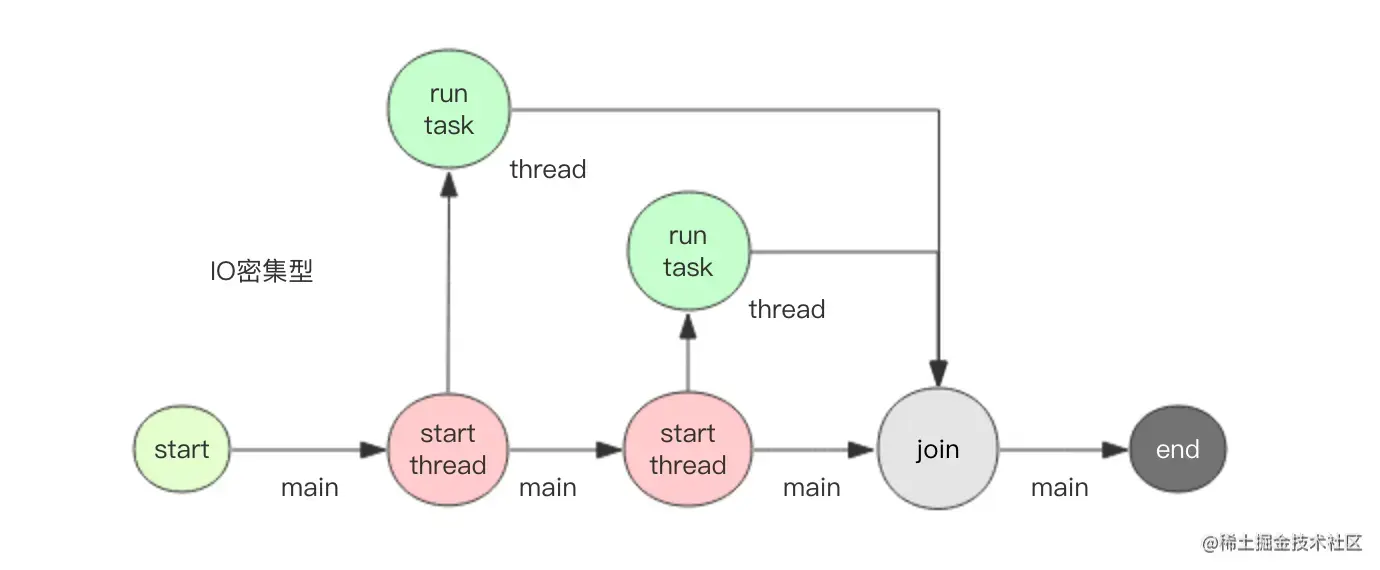Multithreading mode is suitable for IO Intensive operations , Here I want to use sleep Let's simulate a slow IO Mission . At the same time, in order to facilitate the preparation of command-line programs , Use here Google fire Open source library to simplify command line parameter processing .

``````# coding: utf8
# t.py

import time
import fire

time.sleep(1)  #  sleep 1s, simulation IO
return index * index  #  Return results

start = time.time()
fs = []  # future list
wait(fs)  #  Wait for the calculation to end
end = time.time()
duration = end - start
s = sum([f.result() for f in fs])  #  Sum up
print "total result=%s cost: %.2fs" % (s, duration)

if __name__ == '__main__':
fire.Fire(run)
Copy code
Copy code ``````

function `python t.py 2 10`, That is to say 2 Thread run 10 A mission , Observe the output

``````thread 123145422131200 square 0thread 123145426337792 square 1

total result=285 cost: 5.02s
Copy code
Copy code ``````

We see that the total cost of the calculation is about 5s, in total sleep 了 10s Shared by two threads , So it is 5s. The reader may ask , Why is the output messy , This is because print Operation is not atomic , It's two consecutive write Operation synthesis , first write Output content , the second write Output line breaks ,write The operation itself is atomic , But in a multithreaded environment , these two items. write Operations are interleaved , So the output is not neat . If the code is slightly modified , take print Change to single write operation , The output is neat ( About write Whether absolute atomicity needs further discussion )

``````#  Split subtasks
time.sleep(1)  #  sleep 1s, simulation IO
import sys
return index * index  #  Return results
Copy code
Copy code ``````

Let's run again `python t.py 2 10`, Observe the output

``````thread 123145438244864 square 0
total result=285 cost: 5.02s
Copy code
Copy code ``````

Next , We change the parameters , Expand to 10 Threads , See how long all the tasks take to complete

``````> python t.py 10 10
total result=285 cost: 1.01s
Copy code
Copy code ``````

You can see 1s All the tasks are completed in . This is the charm of multithreading , Multiple IO Parallelization of operations , Reduce overall processing time .

## Multi process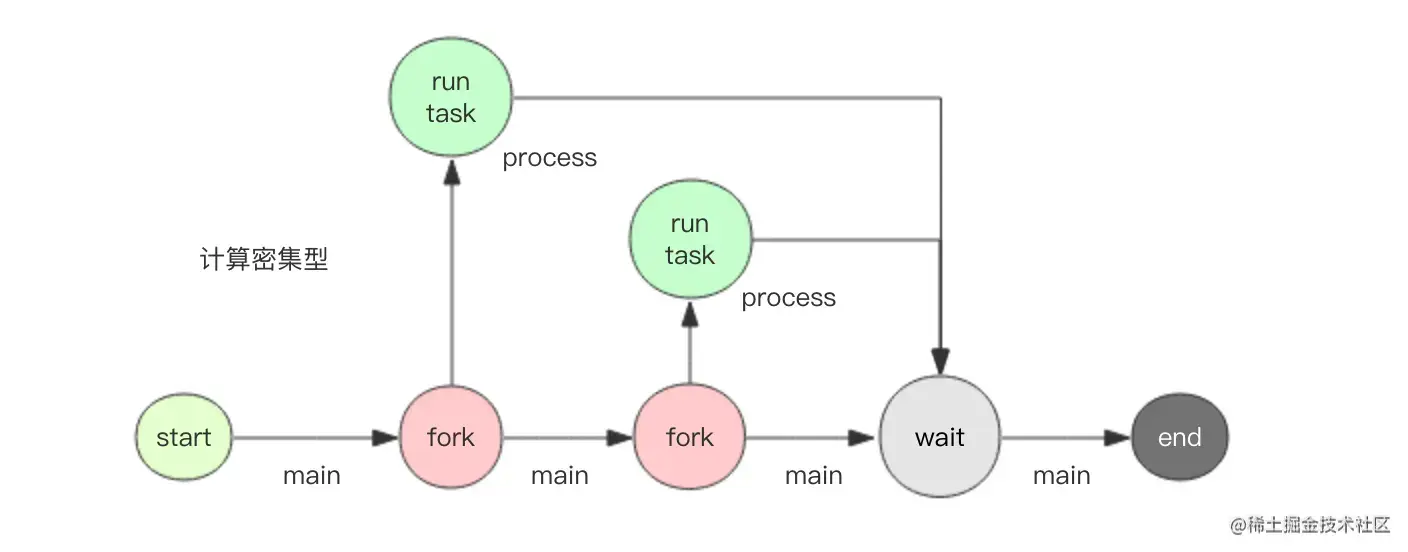Compared with multithreading, it is suitable for processing IO Intensive task , Multi process is suitable for computing intensive systems . Next, let's simulate computing intensive tasks . My PC has 2 Core , You can experience the advantages of multi-core computing .

How to simulate this intensive computing task , We can use the PI formula .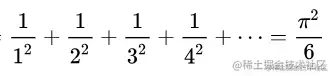By expanding the length of the series n, You can approach PI infinitely . When n When I was very old , The calculation will be slow , Now CPU Will always be busy , This is exactly what we expect .

good , Write the code of multi process parallel computing

``````# coding: utf8
# p.py

import os
import sys
import math
import time
import fire
from concurrent.futures import ProcessPoolExecutor, wait

#  Calculate the PI according to the formula
s = 0.0
for i in range(n):
s += 1.0/(i+1)/(i+1)
pi = math.sqrt(6*s)
# os.getpid You can get the sub process number
sys.stdout.write("process %s n=%d pi=%s\n" % (os.getpid(), n, pi))
return pi

def run(process_num, *ns):  #  Enter multiple n value , Divide into multiple subtasks to calculate the results
#  Instantiate process pool ,process_num A process
executor = ProcessPoolExecutor(process_num)
start = time.time()
fs = []  # future list
for n in ns:
wait(fs)  #  Wait for the calculation to end
end = time.time()
duration = end - start
print "total cost: %.2fs" % duration
executor.shutdown()  #  Destroy process pool

if __name__ == '__main__':
fire.Fire(run)
Copy code
Copy code ``````

From the code, we can see that the multi process mode is not much different from multithreading in code writing , Just change a class name , Everything else is the same . This is also concurrent The charm of Library , The multithreading and multiprocessing model are abstracted out of the same use interface .

Let's run it `python p.py 1 5000000 5001000 5002000 5003000`, All in all 4 Time pi, Only one process . Observe the output

``````process 96354 n=5000000 pi=3.1415924626
process 96354 n=5001000 pi=3.14159246264
process 96354 n=5002000 pi=3.14159246268
process 96354 n=5003000 pi=3.14159246272
total cost: 9.45s
Copy code
Copy code ``````

It can be seen that with n The increase of , The result is getting closer to pi , Because only one process is used , So the task is executed serially , It took about 9.5s.

Next, add another process , Observe the output

``````> python p.py 2 5000000 5001000 5002000 5003000
process 96529 n=5001000 pi=3.14159246264
process 96530 n=5000000 pi=3.1415924626
process 96529 n=5002000 pi=3.14159246268
process 96530 n=5003000 pi=3.14159246272
total cost: 4.98s
Copy code
Copy code ``````

In terms of time consumption, it is close to 1 And a half , It shows that multi process does play the effect of computing parallelization . Now if you use top Command to observe the of the process CPU Usage rate , The difference between these two processes is CPU The utilization rate is close to 100%.

If we add more 2 A process , Can we continue to compress the computing time

``````> python p.py 4 5000000 5001000 5002000 5003000
process 96864 n=5002000 pi=3.14159246268
process 96862 n=5000000 pi=3.1415924626
process 96863 n=5001000 pi=3.14159246264
process 96865 n=5003000 pi=3.14159246272
total cost: 4.86s
Copy code
Copy code ``````

It seems that we can't continue to save time , Because only 2 A computing core ,2 One process is enough to drain them , Even with more progress, there is only 2 Two computing cores are available .

## Deep principle

concurrent It's very simple to use , But the internal implementation is not well understood . Before delving into the internal structure , We need to understand Future This object . In the previous example ,executor Submit (submit) A will be returned after the task Future object , It represents a pit of results , When the task has just been submitted , The pit is empty , Once the child thread runs, the task ends , Will plug the results of the operation into the pit , The main thread can pass Future Object to get this result . In a nutshell ,Future Object is the medium through which the main thread and the child thread communicate .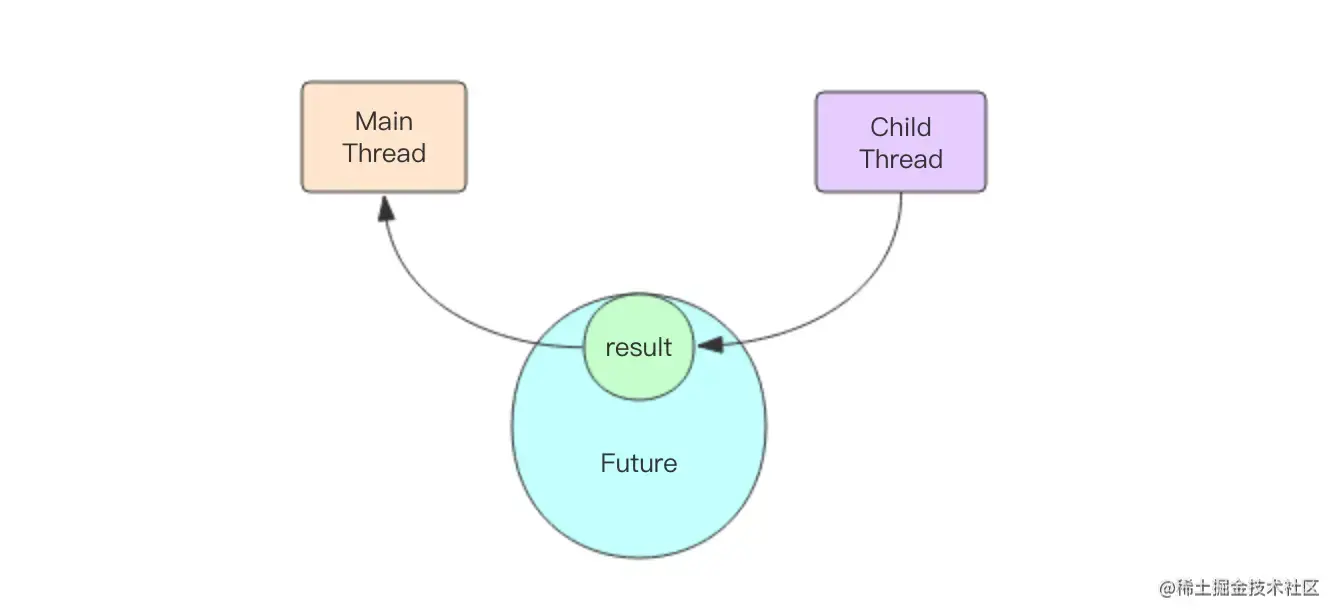Future The internal logic of the object is simpler and can be represented by the following code

``````class Future(object):

def __init__(self):
self._condition = threading.Condition()  #  Condition variables,
self._result = None

def result(self, timeout=None):
self._condition.wait(timeout)
return self._result

def set_result(self, result):
self._result = result
self._condition.notify_all()
Copy code
Copy code ``````

The main thread gets this after it inserts the task into the thread pool Future object , Its internal _result Or empty? . If the main thread calls result() Method get results , Will block on the condition variable . If the calculation task of the child thread is completed, it will immediately call set_result() Method to populate the results into future object , And wake up the thread blocking on the condition variable , That is, the main thread . At this time, the main process immediately wakes up and returns the results normally .

The interaction between main thread and sub thread is divided into two parts , The first part is how the main thread passes the task to the child thread , The second part is how the child thread passes the result to the main thread . The second part has been said through Future Object to complete . How did the first part do it ？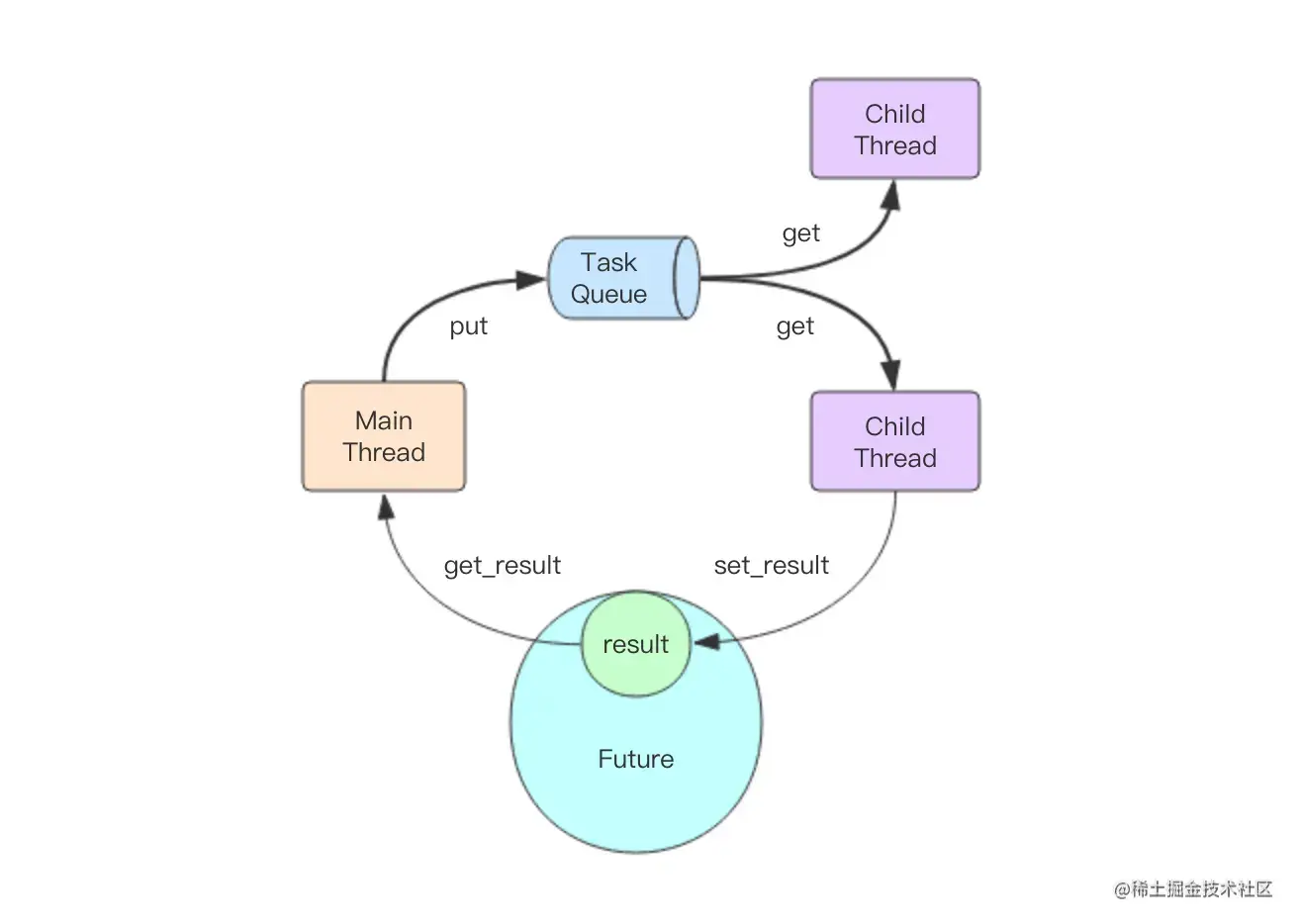As shown in the figure above , The secret lies in this queue , The main thread passes tasks to multiple child threads through a queue . Once the main thread pushes the task into the task queue , The sub threads will start competing , Finally, only one thread can grab the task , And execute immediately , After execution, put the results into Future Object completes the whole execution process of this task .

concurrent There is a major design problem with the thread pool , That is, the task queue is unbounded . If the queued producer task is producing too fast , Thread pool consumption is too slow to handle , Tasks pile up . If the accumulation continues , Memory will continue to grow until OOM, All the tasks accumulated in the task queue are completely lost . Users must pay attention to this when using , And make proper control .

## Internal structure of process pool

The internal structure of the process pool is complex , even concurent The authors of the library themselves find it particularly complex , So I drew a picture in the code ascii Figure to explain the internal structure of the model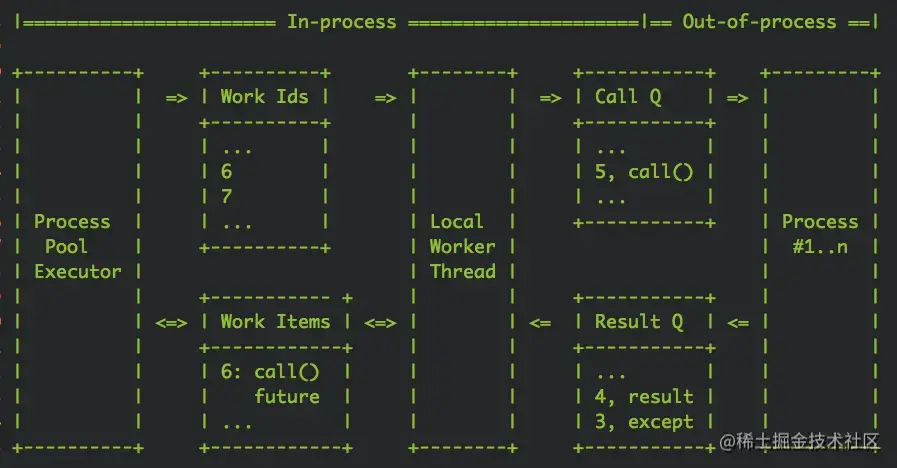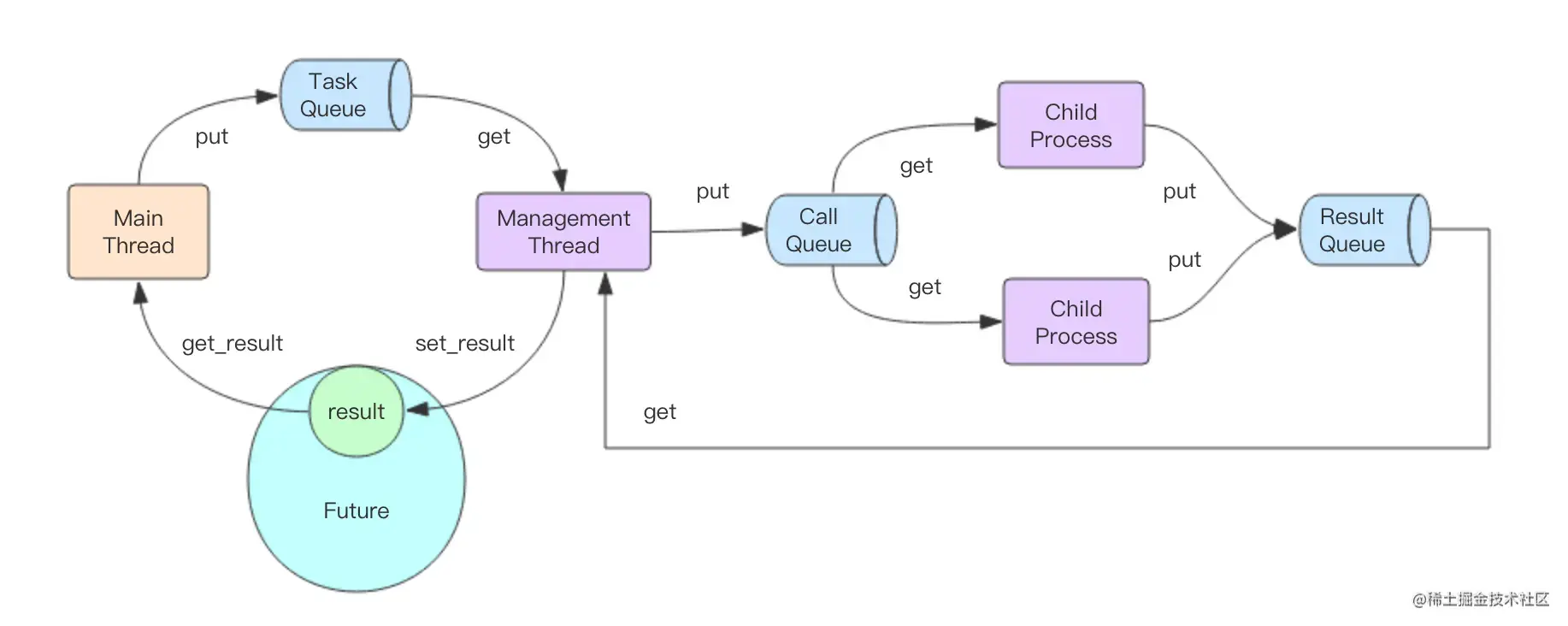I don't think the author's picture is easy enough to understand , So I drew a separate picture , Please carefully combine the above two pictures , Let's go through the complete task processing process .

1. The mainline crams tasks into TaskQueue( Normal memory queue ), Get Future object
3. Subprocesses from CallQueue Compete for tasks in
4. The subprocess inserts the processing results into ResultQueue( Distributed cross process queue )
5. Management thread from ResultQueue Get results in , Stuff in Future object
6. The main thread is from Future Get the result from the object

This complex process involves 3 A queue , There are additional management threads in the middle . Then why did the author design so complex , What's the advantage of such a design ？

First , Let's look at the left half of this picture , It is not much different from the processing flow of thread pool , The only difference is that there is only one management thread , The child threads of the thread pool will have multiple . This design can make the use of multi process model and multi thread model consistent , This is why there is no difference between the two models —— Through the middle management thread, the multi process interaction logic behind it is hidden .

Then let's look at the right half of this picture , The management thread interacts with child processes through two queues , Both queues are cross process queues (multiprocessing.Queue).CallQueue It is a single producer and multiple consumers ,ResultQueue It's a multi producer single consumer .

CallQueue It's a bounded queue , Its upper limit is written dead in the code as 「 Number of child processes +1」. If the child processes can't handle it ,CallQueue It will be full , The management thread will stop stuffing data into it . But there are also the same problems as thread pool ,TaskQueue It's a boundless line , Its content doesn't matter whether consumers are continuing ( Manage threads ) consumption ,TaskQueue Will continue to grow indefinitely , So it will eventually lead to OOM.

## Cross process queue

The cross process queue in the process pool model uses multiprocessing.Queue Realized . What are the internal details of the cross process queue , What high technology is used to realize it

The author read carefully multiprocessing.Queue Source code discovery for , It uses an anonymous socket sockerpair To complete cross process communication ,socketpair and socket The difference is socketpair No ports are needed , You don't need to go through the network protocol stack , Direct cross process communication through the socket read-write buffer of the kernel .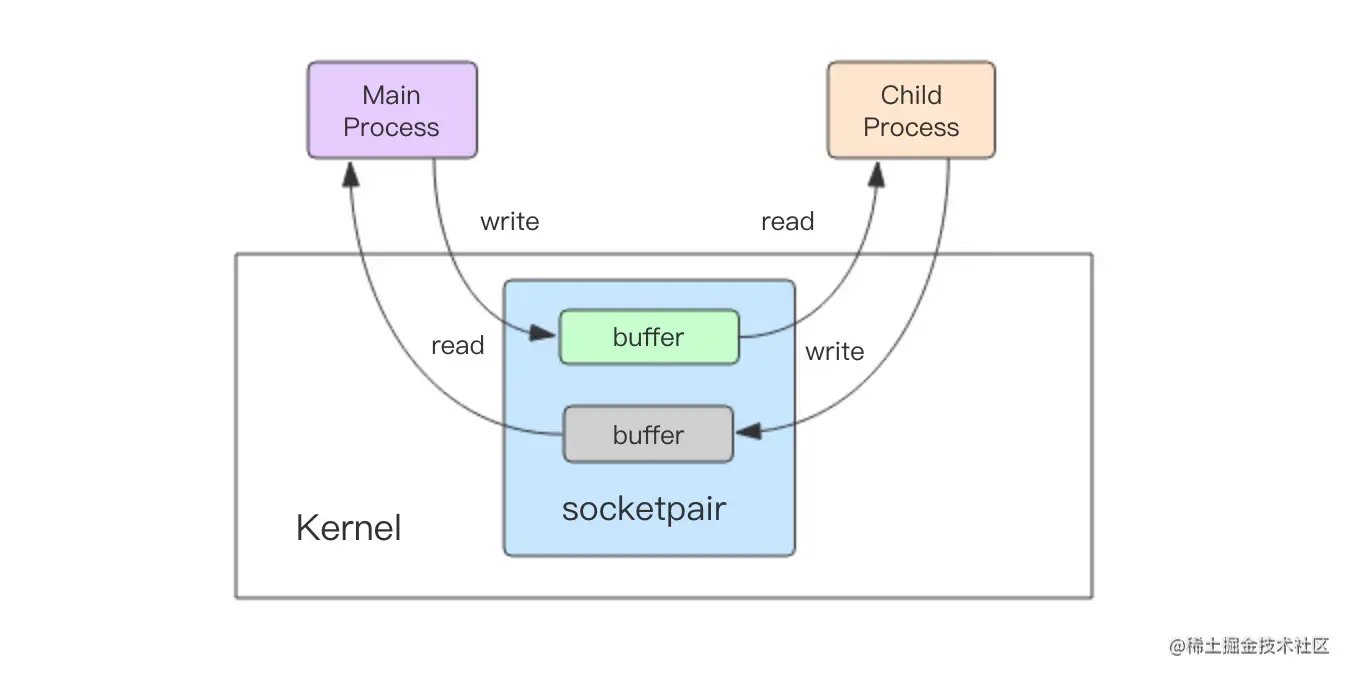When the parent process wants to pass tasks to the child process , First use pickle Serialize the task object into a byte array , Then pass the byte array through socketpair The write descriptor is written to the kernel buffer in . The subprocess can then start from buffer Byte array read from , And then use pickle Deserialize the byte array to get the task object , So we can finally perform the task . In the same way, the process passes the result to the parent process and follows the same process , It's just that the socketpair yes ResultQueue Anonymous socket created internally .

multiprocessing.Queue It supports duplex communication , Data flow can be parent to child , It can also be from son to father , But in concurrent Only simplex communication is used in the implementation of process pool .CallQueue From father to son ,ResultQueue From son to father .

## summary

concurrent.futures The frame is very easy to use , Although the internal implementation mechanism is extremely complex , Readers can use it directly without fully understanding the internal details . However, it should be noted that the task queue inside the thread pool or process pool is unbounded , We must avoid consumers' untimely processing and the continuous rise of memory .